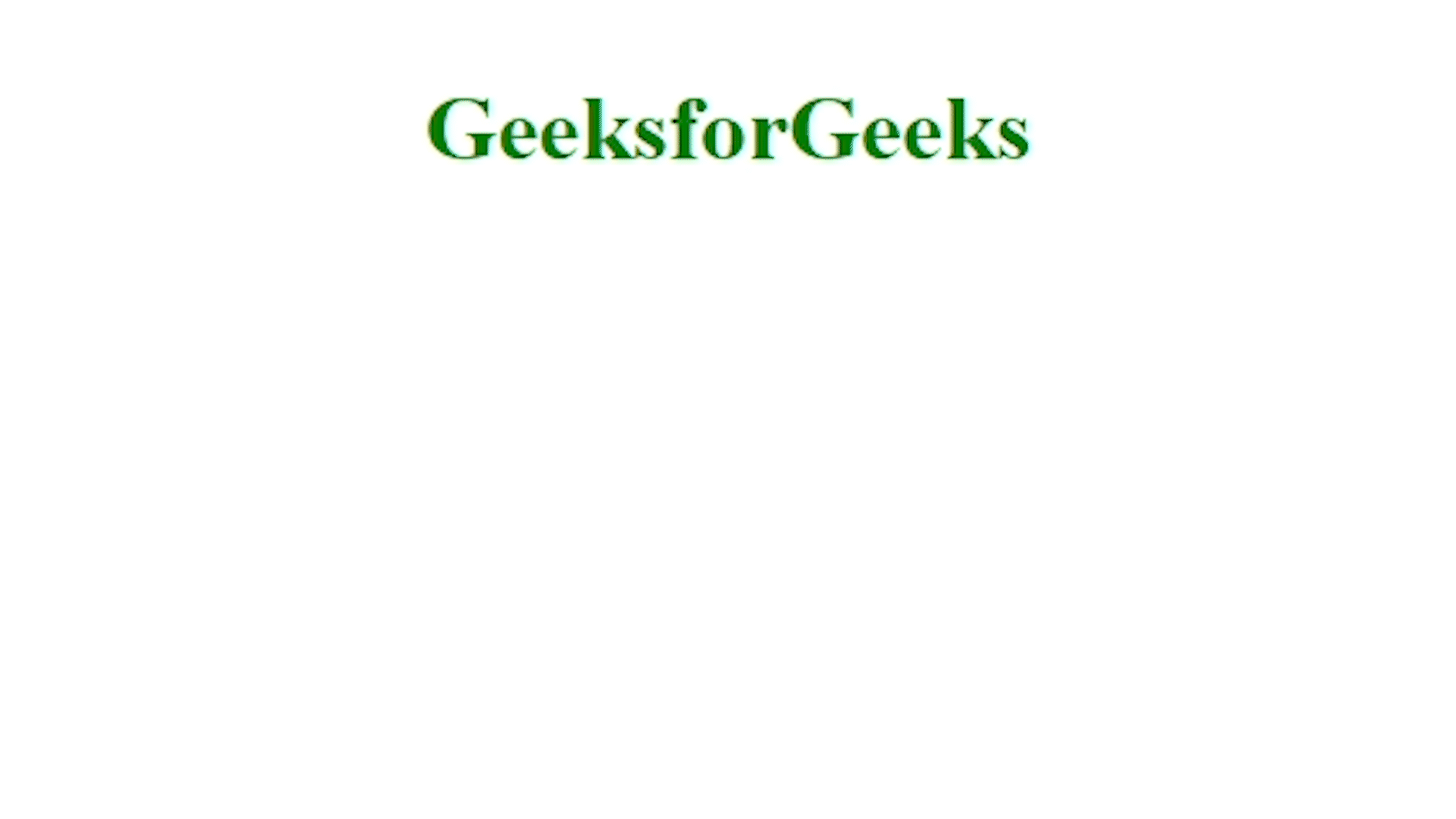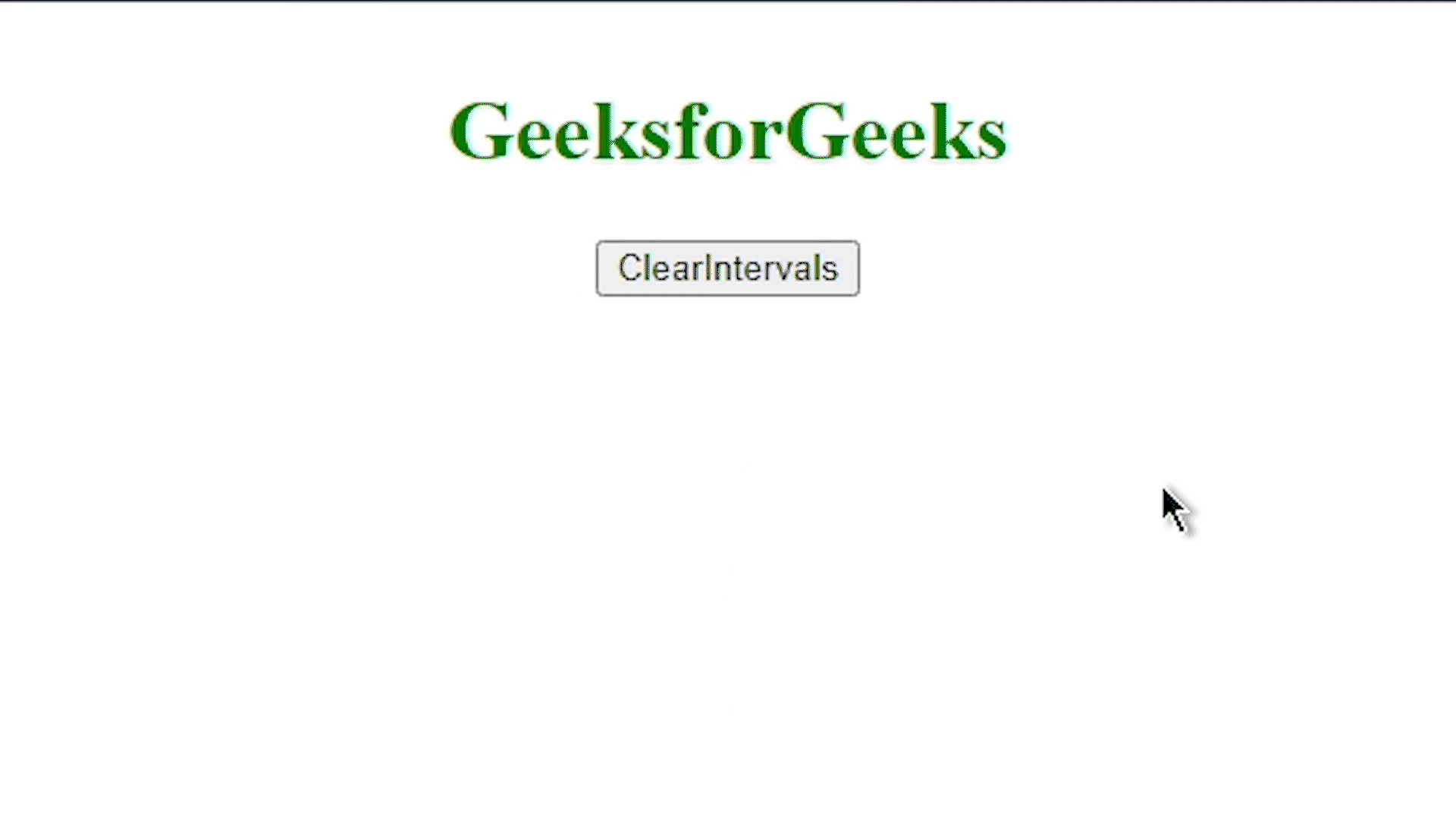# How to clear setInterval without knowing the ID in jQuery ?

In this article, we are going to learn how can we clear setInterval without knowing its ID using jQuery. First of all, let’s briefly understand about setInterval. This method is used for executing any specific function or some section of code at a particular period of time So that we don’t have to use that over and over. When we use setInterval we are also obliged to use the clear interval method, otherwise, that setInterval will run its code till the memory runs out of space.

Syntax:

`let ID = setInterval(function, <delay>);`

When we use the upper syntax, the ID returns an integer value that is called the ID of setInterval, and this value will be further used for clearing the setInterval. Now we are going to look at two examples in which we will clear the setInterval using ID and without knowing the id of the setInterval, that’s this article’s main purpose.

Approach: When we use setinterval method, it automatically generated an id, since we don’t know what it is so we will be using a general looping method to clear the interval, we will run a loop from 0 to a certain long number so that every interval contains between that will be clear. See Example 2, below.

Example 1: In this example, we will use the setInterval method to print the counting and we will clear the interval using the ID.

## HTML

 `` `<``html` `lang``=``"en"``>` `<``head``>` `    ``<``script` `src``=` `"https://code.jquery.com/jquery-3.3.1.min.js"` `            ``integrity``=` `"sha256-FgpCb/KJQlLNfOu91ta32o/NMZxltwRo8QtmkMRdAu8="` `            ``crossorigin``=``"anonymous"``>` `     ``` `` `<``style``>` `    ``#count {` `        ``display: flex;` `        ``align-items: center;` `        ``justify-content: center;` `        ``flex-direction: column;` `    ``}` `` `<``body``>` `    ``<``div` `id``=``"count"``>` `        ``<``h1` `style``=``"color: green;"``>` `          ``GeeksforGeeks` `        ``` `    ``` `` `<``script``>` `    ``\$(document).ready(function () {` `        ``let count = 0;` `        ``let id = setInterval(function () {` `            ``count++;` `            ``\$('#count').append(count + '<``br``>');`   `            ``// clearing the interval` `            ``if (count == 5) {` `                ``clearInterval(id);` `            ``}` `        ``}, 500);` `    ``});` `` ``

Output:Example 2: In this example, we won’t be using ID for clearing the interval, we will use clear interval and loop it through all possible id of the interval, let’s see the example for better understanding.

## HTML

 `` `<``html` `lang``=``"en"``>` `<``head``>` `    ``<``script` `src``=` `"https://code.jquery.com/jquery-3.3.1.min.js"` `            ``integrity``=` `"sha256-FgpCb/KJQlLNfOu91ta32o/NMZxltwRo8QtmkMRdAu8="` `            ``crossorigin``=``"anonymous"``>` `     ``` `` `<``style``>` `    ``#count {` `        ``display: flex;` `        ``align-items: center;` `        ``justify-content: center;` `        ``flex-direction: column;` `    ``}` `` `<``body``>` `    ``<``div` `id``=``"count"``>` `        ``<``h1` `style``=``"color: green;"``>GeeksforGeeks` `        ``<``button` `id``=``"clear"``>ClearIntervals` `    ``` `` `<``script``>` `    ``\$(document).ready(function () {` `        ``let count = 0;` `        ``let id = setInterval(function () {` `            ``count++;` `            ``\$('#count').append(count + '<``br``>');` `        ``}, 500);`   `        ``\$('#clear').click(function () {` `            ``for (let i = 1; i < ``99999``; i++)` `                ``clearInterval(i);` `        ``});` `    ``});` `` ``

Output:Whether you're preparing for your first job interview or aiming to upskill in this ever-evolving tech landscape, GeeksforGeeks Courses are your key to success. We provide top-quality content at affordable prices, all geared towards accelerating your growth in a time-bound manner. Join the millions we've already empowered, and we're here to do the same for you. Don't miss out - check it out now!